# Astropy - Modeling¶

In :
import numpy as np
%matplotlib inline
import matplotlib.pyplot as plt

In :
from astropy.modeling import models, fitting


## Setting up models¶

In :
g = models.Gaussian1D(amplitude=1.2, mean=0.9, stddev=0.5)
print(g)

Model: Gaussian1D
Inputs: ('x',)
Outputs: ('y',)
Model set size: 1
Parameters:
amplitude mean stddev
--------- ---- ------
1.2  0.9    0.5


Model parameters can be accessed as attributes:

In :
g.amplitude

Out:
Parameter('amplitude', value=1.2)
In :
g.mean

Out:
Parameter('mean', value=0.9)
In :
g.stddev

Out:
Parameter('stddev', value=0.5)

and can also be updated via those attributes:

In :
g.amplitude = 0.8
g.amplitude

Out:
Parameter('amplitude', value=0.8)

Models can be evaluated directly (so they are useful without even fitting):

In :
g(1.3)

Out:
0.5809192296589527
In :
x = np.linspace(-5, 5, 1000)
plt.plot(x, g(x))

Out:
[<matplotlib.lines.Line2D at 0x10ab72d68>]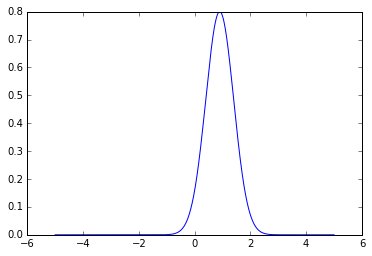## Fitting models to data¶

We start off by generating fake data:

In :
np.random.seed(0)
x = np.linspace(-5., 5., 200)
y = 3 * np.exp(-0.5 * (x - 1.3)**2 / 0.8**2)
y += np.random.normal(0., 0.2, x.shape)
plt.plot(x, y, 'ko')

Out:
[<matplotlib.lines.Line2D at 0x10ac23ba8>]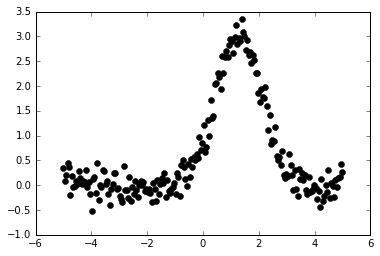We set up a fitter:

In :
fitter = fitting.LevMarLSQFitter()


We now fit the data using a trapezoid model and a Gaussian

In :
t_init = models.Trapezoid1D(amplitude=1., x_0=0., width=1., slope=0.5)
t = fitter(t_init, x, y)

g_init = models.Gaussian1D(amplitude=1., mean=0, stddev=1.)
g = fitter(g_init, x, y)


Finally we can plot the data and the two models

In :
plt.figure(figsize=(8,5))
plt.plot(x, y, 'ko')
plt.plot(x, t(x), 'b-', lw=2, label='Trapezoid')
plt.plot(x, g(x), 'r-', lw=2, label='Gaussian')
plt.xlabel('Position')
plt.ylabel('Flux')
plt.legend(loc=2)

Out:
<matplotlib.legend.Legend at 0x10c0b3898>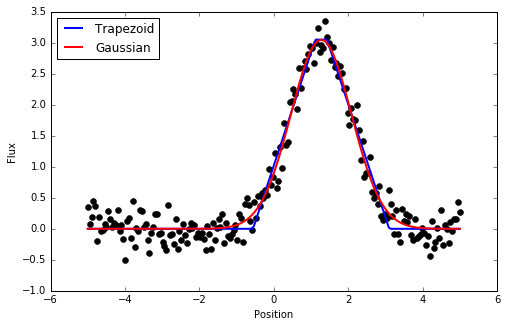## Multi-dimensional models¶

The modeling framework is not restricted to 1-d models and datasets. To demonstrate this, we create a 2-d dataset:

In :
np.random.seed(0)
y, x = np.mgrid[:128, :128]
z = 2. * x ** 2 - 0.5 * x ** 2 + 1.5 * x * y - 1.
z += np.random.normal(0., 0.1, z.shape) * 50000.
plt.imshow(z, origin='lower', interpolation='nearest', vmin=-1e4, vmax=5e4)

Out:
<matplotlib.image.AxesImage at 0x10c22d400>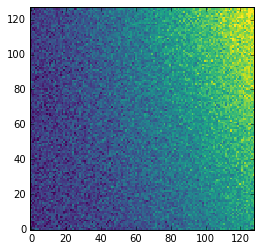As before, we set up a fitter:

In :
fitter = fitting.LinearLSQFitter()


We create the initial model:

In :
p_init = models.Polynomial2D(degree=2)


and we can now fit the model to the data:

In :
p = fitter(p_init, x, y, z)


We can now look at the best model

In :
plt.imshow(p(x, y), origin='lower', interpolation='nearest', vmin=-1e4, vmax=5e4)

Out:
<matplotlib.image.AxesImage at 0x10ca6da20>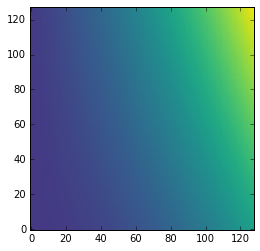and the residual

In :
plt.imshow(z - p(x, y), origin='lower', interpolation='nearest', vmin=-1e4, vmax=5e4)

Out:
<matplotlib.image.AxesImage at 0x10de39fd0>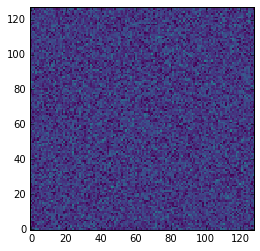## Compound models¶

In some cases, we may want to fit multiple models at the same time (for example two Gaussians, or a background polynomial and a Gaussian, etc.). This is now possible in Astropy 1.0 and later. As before we start off by generating synthetic data:

In :
np.random.seed(42)
g1 = models.Gaussian1D(1, 0, 0.2)
g2 = models.Gaussian1D(2.5, 0.5, 0.1)
x = np.linspace(-1, 1, 200)
y = g1(x) + g2(x) + np.random.normal(0., 0.2, x.shape)
plt.plot(x, y, 'ko')

Out:
[<matplotlib.lines.Line2D at 0x10c1eed30>]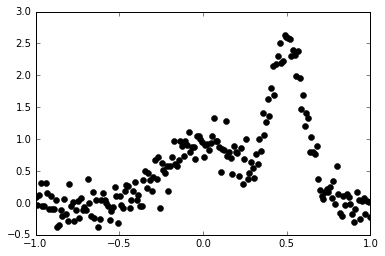Now we fit this with a combination of two Gaussians:

In :
gg_init = models.Gaussian1D(1, 0, 0.1) + models.Gaussian1D(2, 0.5, 0.1)
fitter = fitting.SLSQPLSQFitter()
gg_fit = fitter(gg_init, x, y)

Optimization terminated successfully.    (Exit mode 0)
Current function value: 6.83285936044
Iterations: 14
Function evaluations: 137


Finally, we can plot the best-fitting model:

In :
# Plot the data with the best-fit model
plt.figure(figsize=(8,5))
plt.plot(x, y, 'ko')
plt.plot(x, gg_fit(x), 'r-', lw=2)
plt.xlabel('Position')
plt.ylabel('Flux')

Out:
<matplotlib.text.Text at 0x10df655f8>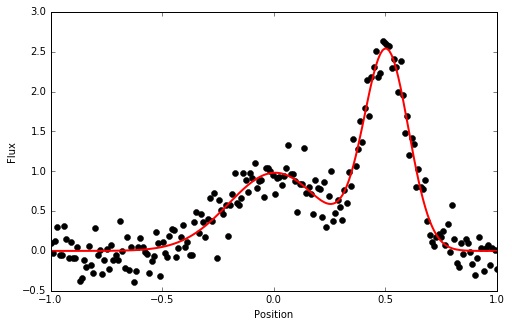## Exercises¶

### Level 1¶

Read in the data from data/fitting_data.txt and try and fit it with a straight line and a parabola, then make a plot of the resulting fits.

### Level 2¶

Download the first FITS spectrum from this page, read it in with astropy.io.fits and try and fit a compound model of a gaussian plus a constant to the spectrum (just assume the points are equally spaced in wavelength space, even if that may not be true). Hint: you may have to play around with setting better initial values for the position of the line.

### Level 3¶

Try and read in an image of a star field, and try fitting a 2D Gaussian to one of the stars.